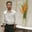Related Tags

html

# How to draw a rectangle using the SVG element in HTMLBehzad Ahmad

### Overview

SVG stands for Scalable Vector Graphics. It is a language which is used to describe the 2D graphics and the XML graphical applications. Then the SVG viewer renders XML. Many web browsers display the SVG just like the png, jpg, and gif.

We can create the rectangle by using the <rect> element:

### Syntax

<rect
x="co-ordinates of x-axis"
y="co-ordinates of y-axis"

width="length"
height="length"

rx="length"
ry="length" >
</rect>


### Parameters

• x: Co-ordinates of the top left x-axis.

• y: Co-ordinates of the top left y-axis.

• width: The width of the rectangle.

• height: The height of the rectangle.

• rx: This defines how much we want to round the x-axis.

• ry: This defines how much we want to round the y-axis.

### Example

Let’s discuss the basic example to see how to make a rectangle:

### Explanation

• Line 4: We define the height and width of the SVG element.

• Line 5: We define the height and width of the rectangle and the color we want to fill in the rectangle.

### Explanation

• Line 4: We define the height and width of the SVG element.

• Line 5: We define the height and width of the rectangle and the amount of roundness of the rectangle and the color we want to fill in the rectangle.

RELATED TAGS

html

CONTRIBUTORBehzad Ahmad
RELATED COURSES

View all Courses

Keep Exploring

Learn in-demand tech skills in half the time